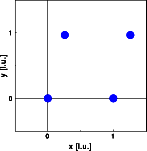Interactive Tutorial about Diffraction Reciprocal space IV: Oblique latticeReciprocal space Reciprocity Square lattice Rectangular lattice Oblique lattice Translation of lattice Rotation lattice Interactive examples 2D crystal builder Planes and HKL's Finite size effect Goto Contents In this example a monoclinic lattice is simulated. The unit cell lengths are identical, the angel between the base vectors is 75 degrees. Two by two unit cells are simulated, each containing one atom at (0 0). This miniature crystal is sufficient in size to illustrate the geometrical relationships. By increasing the size, we will just decrease the FWHM of the Bragg reflections. The pictures below show the atoms and the corresponding Fourier transform. Click on the pictures for a full screen version.The reciprocal space geometry (right image) corresponds to that of the real space. The reciprocal base vectors are of equal length, but the angle between the base vectors is 180 - 75 = 105 degrees. The vertical reciprocal base vector is normal to the horizontal direct space vector, while the second reciprocal base vector (to the right and slightly down) is normal to the other real space vector (up and slightly right). © Th. Proffen and R.B. Neder, 2003